## Matrices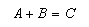Addition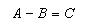Subtraction: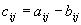Multiplication:Multiplication with a number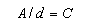Division: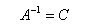Inverse: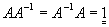Determinante: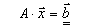Multiplication with vector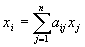Solution of linear equationsystem (only if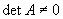)Rotation matrix around vektorand angle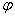### Special functions

 A->0 All values of A are set to 0. A->1 All diagonal values of A are set to 1, the other to 0. A->AA will be inverted. A->A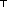A will be transposed: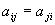A->-A Changes the sign of all values of A.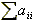Sum of diagonal elements det A Determinante Rg A Quality of A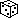A Fills the matrix A with random values.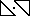C All values are set to 0. 1 All diagonal values are set to 1, the other to 0